Disclosure: This post may contain affiliate links, meaning when you click the links and make a purchase, we receive a commission.

# How to auto populate date in Excel when cell is updated

In this article, I will show you how to auto-populate date in Excel when a cell is updated. I will show the whole process using Excel VBA. I will also show how you can modify the code for your specific purposes.

Sometimes several people work on a worksheet and anyone can update the worksheet anytime. So, the worksheet owner might want to see the last updates with the update date and time.

## Auto-populating dates in cells using Excel VBA (for the whole column)

Take a look at the following dynamic image. You see when I put some value in a cell of column A, adjacent column B cell show the date and time I am changing the cell.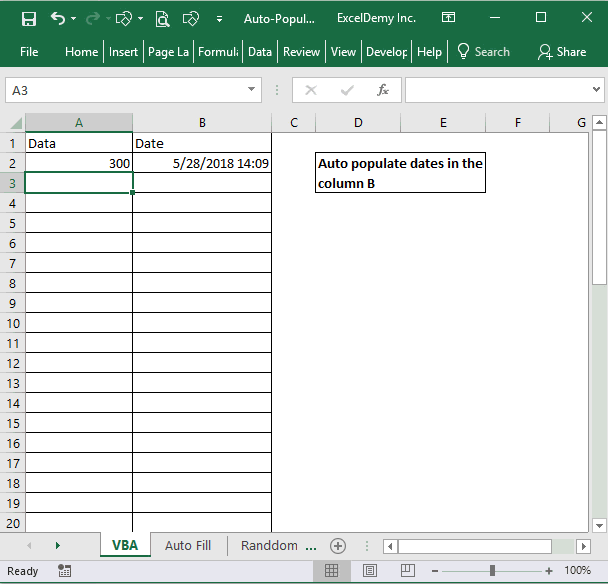You update a cell and the adjacent cell shows the update date and time.

### VBA Code and step by step procedure

 Source code```Private Sub Worksheet_Change(ByVal Target As Range)
' Auto Date
Dim Cell As Range
For Each Cell In Target
If Cell.Column = Range("A:A").Column Then
If Cell.Value <> "" Then
Cells(Cell.Row, "B").Value = Now
Else
Cells(Cell.Row, "B").Value = ""
End If
End If
Next Cell
End Sub```

This is the VBA code that I am going to use. Let’s show you where to put this code.

1) Click on the Developer tab. And then click on the Visual Basic command in the Code window.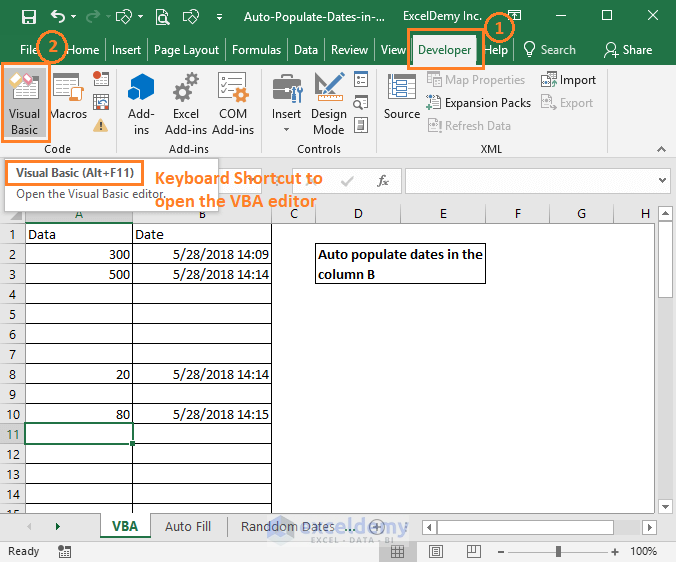To open Excel VBA editor go to Developer tab and then click on the Visual Basic command in the Code window.

2) Microsoft VBA code editor will open like the following image. The keyboard shortcut to open this window is ALT + F11.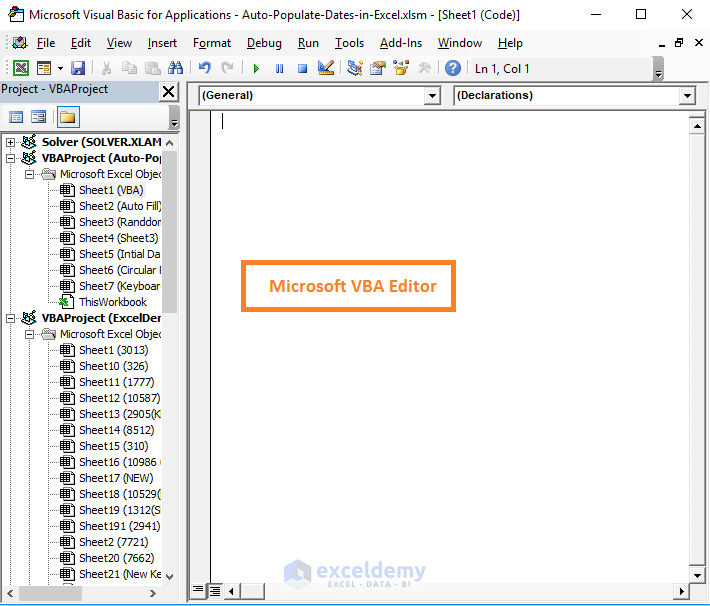When you will open the VBA editor, it might show blank or the existing Macro codes already available in the same workbook or from any workbook open in your Windows system.

3) Double click on the worksheet on which you want to apply our code. So, you will find an editor that looks like this.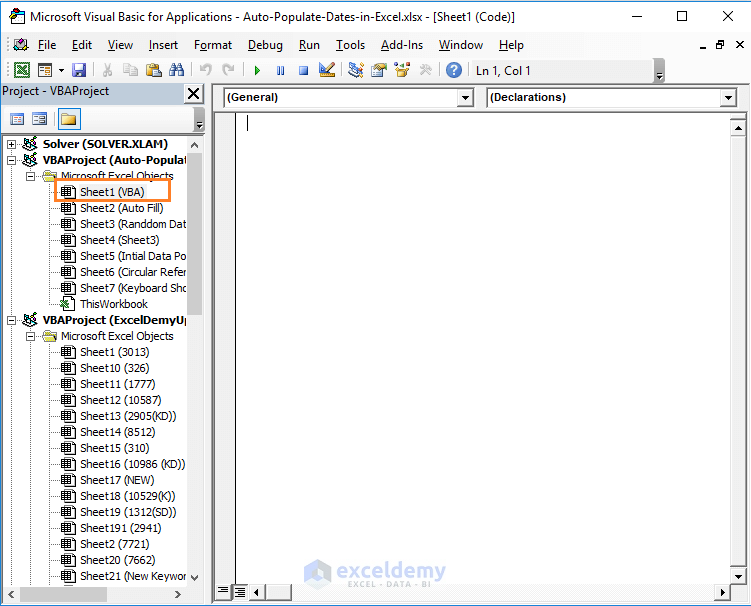To open the Sheet1 worksheet (named as VBA) related code editor, double-click on the Sheet1 (VBA) on the left side of the editor.

4) Click the drop-down part of the (General) and choose Worksheet.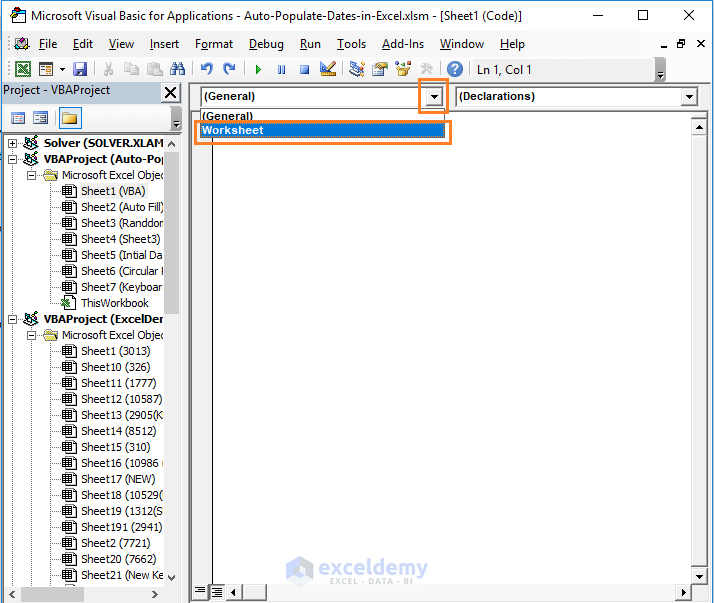Select Worksheet from the drop down (on the left side). When you will select Worksheet, all worksheet related pre-built functions will be available to you.

5) In the same way, from the right-side tab, choose Change option. You will find that two codes are appearing in the code editor. Select and delete the lower part code.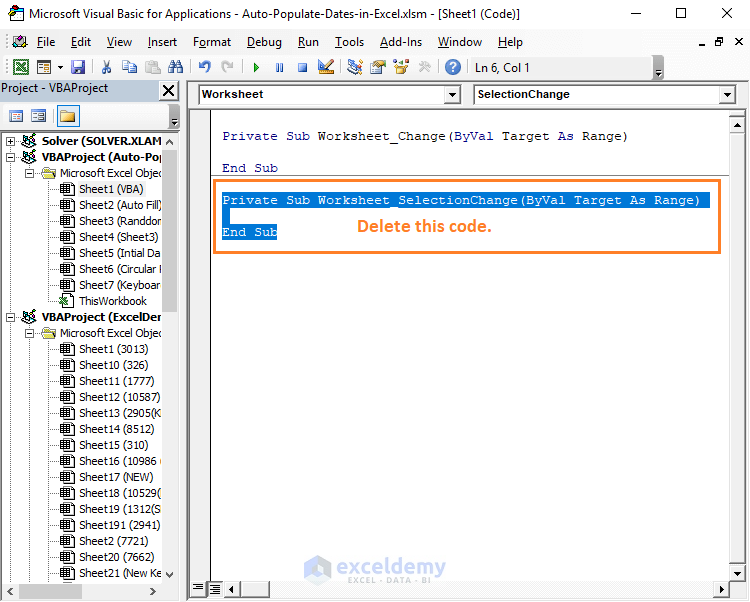Delete the SelectionChange related code from the editor. It is not necessary. I am suggesting to delete this part just for making the editor clean for working.

6) Now insert the code below between these lines:

Private Sub Worksheet_Change(ByVal Target As Range)

‘ Insert code here

End Sub

 Source code```' Auto Date
Dim Cell As Range
For Each Cell In Target
If Cell.Column = Range("A:A").Column Then
If Cell.Value <> "" Then
Cells(Cell.Row, "B").Value = Now
Else
Cells(Cell.Row, "B").Value = ""
End If
End If
Next Cell```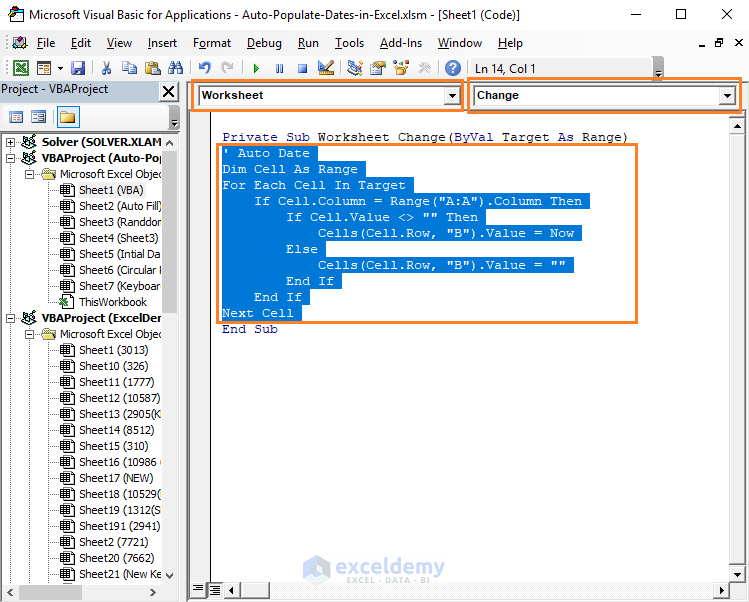When Worksheet is selected, Change is selected, input the above code in the function area.

7) We are done. Now save your workbook pressing CTRL + S or from the File tab. You will see the following Microsoft message. This message will show if your workbook is saved with .xlsx extension instead of .xlsm extension. Your workbook now has VBA code, so it cannot be saved with .xlsx extension.

Select No as we want to save the workbook as a macro-enabled file type.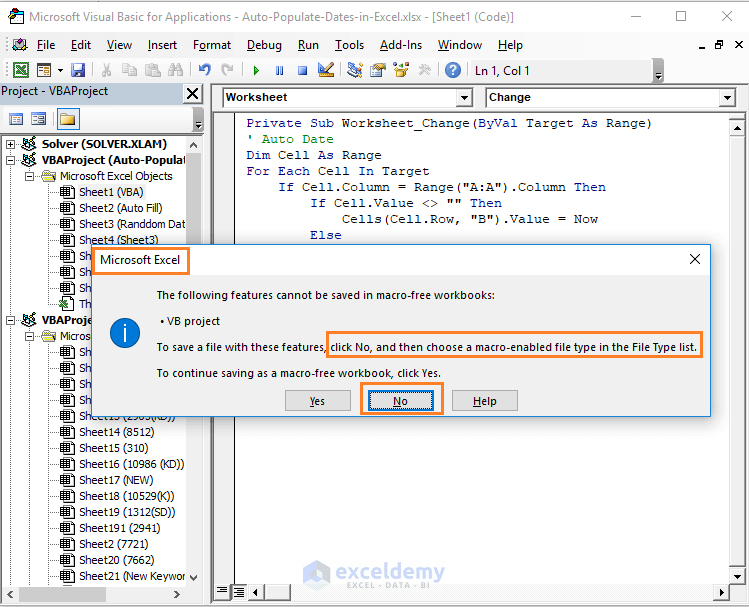You cannot save a workbook with macro codes with .xlsx extension.

8) Choose Excel Macro-Enabled Workbook (.xlsm) option from the Save as type menu and save the file newly.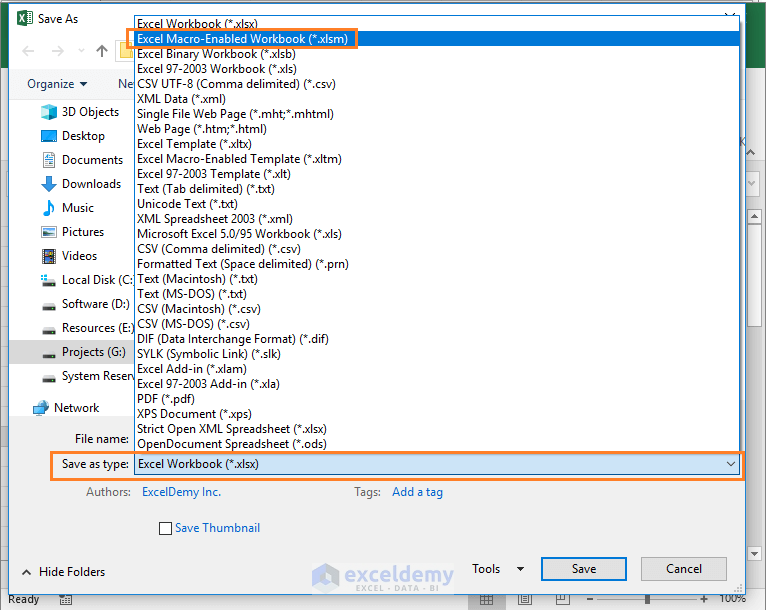Choose the Excel Macro-Enabled Workbook (.xlsm) from the options.

So, this is the whole procedure to auto-populate a cell when a change occurs in the adjacent cell.

### Now the big question. How will you use this code for your specific purpose?

Say you want to use this code for another worksheet and for specific cell ranges. Like the image below.

You want to use this code for VBA2 worksheet and for the columns C and D.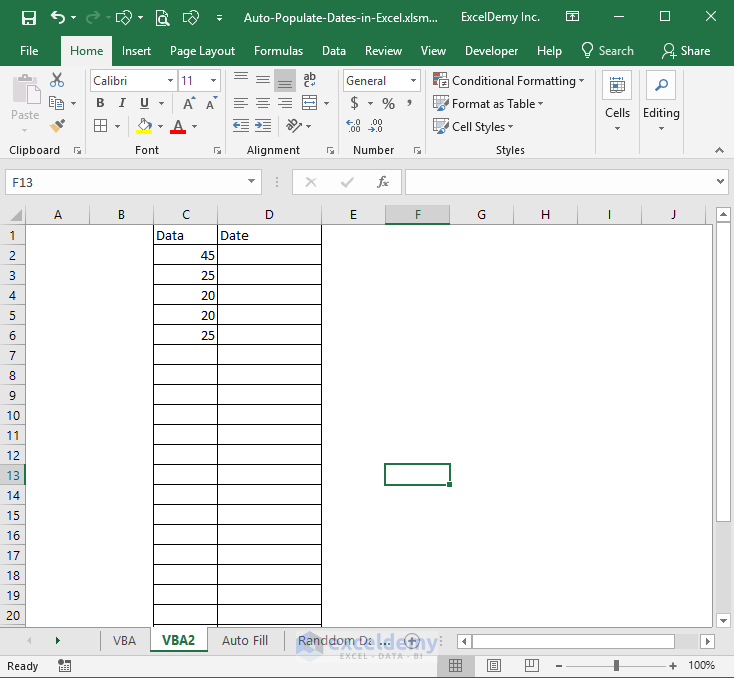We will now modify the above code for different cell ranges.

Here are the steps:

1) Open the Visual Basic editor and you might find that already the previous code is showing while the previous worksheet is selected.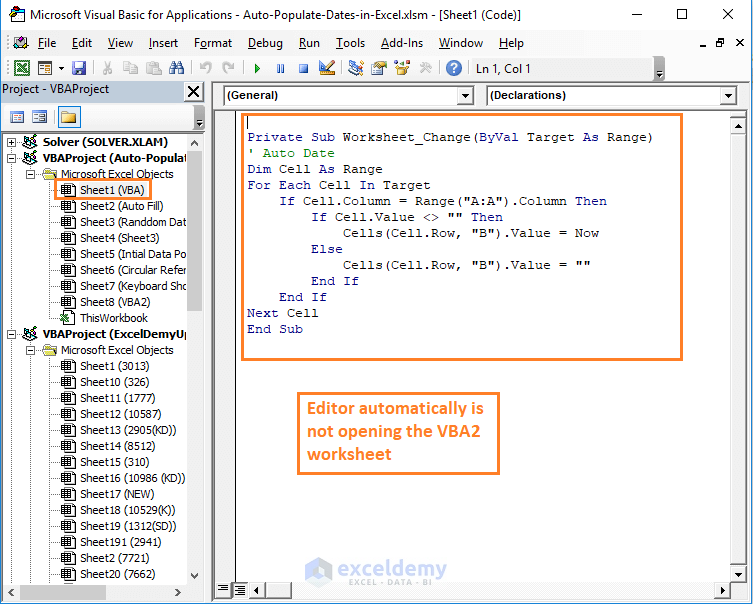Excel VBA editor will show the existing codes and related worksheet when you will open again the VBA editor.

2) Double click on the worksheet on which you want to apply your VBA code. On the right side, choose Worksheet on the left and on the right choose the Change option from the drop-down.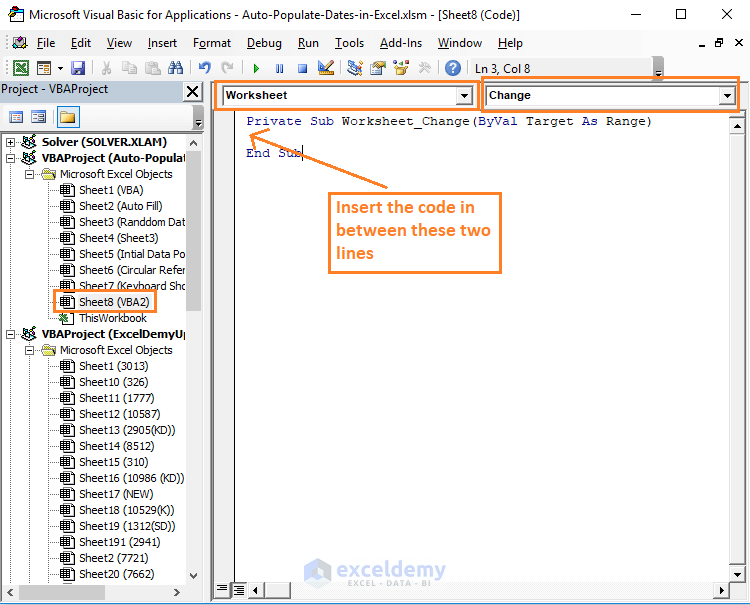To select code editor for a specific worksheet, you have to double-click on it.

3) Now use the following code:

 Source code```' Auto Date
Dim Cell As Range
For Each Cell In Target
If Cell.Column = Range("C:C").Column Then
If Cell.Value <> "" Then
Cells(Cell.Row, "D").Value = Now
Else
Cells(Cell.Row, "D").Value = ""
End If
End If
Next Cell```

I am showing the places where you will make the changes.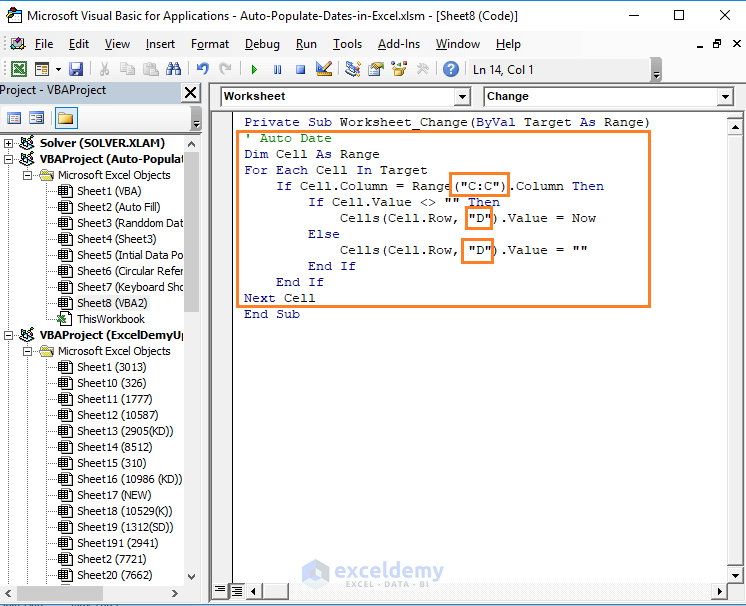See where I am making the changes: C:C and D.

## Auto-populating dates in some specific cells (not the entire column)

Look at the following spreadsheet. Say, in this worksheet, people can only update data on cell range D3: D22 and adjacent cells on column E will record the date of last changes.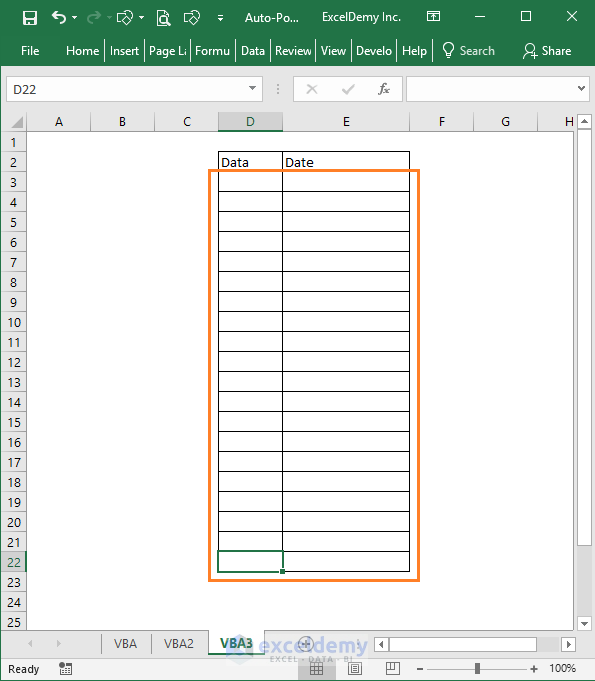This time we are going to show how to auto-populate dates some specific cells (D3: E22), not the entire column.

1) Use the following code:

 Source code```Private Sub Worksheet_Change(ByVal Target As Excel.Range)
With Target
If .Count > 1 Then Exit Sub
If Not Intersect(Range("D3:D22"), .Cells) Is Nothing Then
Application.EnableEvents = False
If IsEmpty(.Value) Then
.Offset(0, 1).ClearContents
Else
With .Offset(0, 1)
.NumberFormat = "dd mmm yyyy hh:mm:ss"
.Value = Now
End With
End If
Application.EnableEvents = True
End If
End With
End Sub```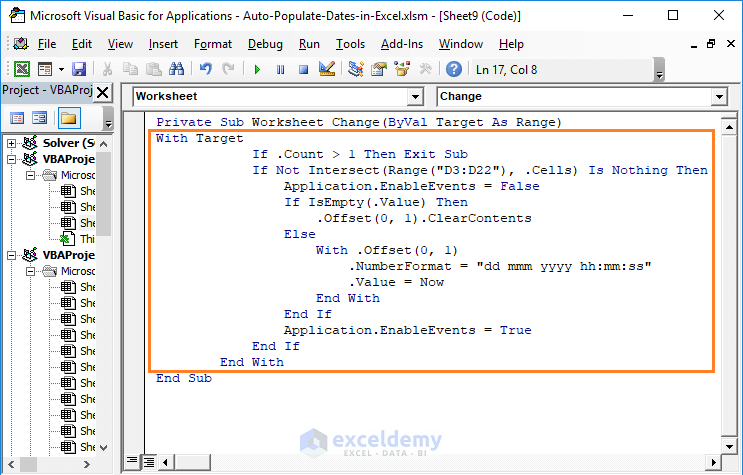Input this code and then see the magic.

2) Here is the result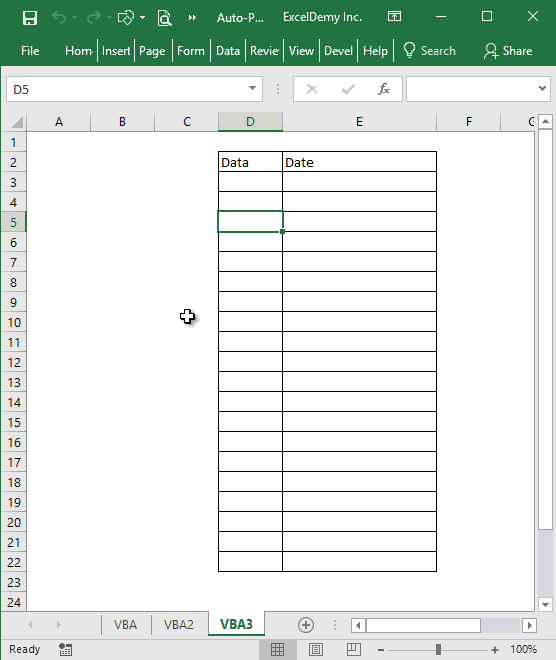Looks good.

This code will impact only the cell range D3: E22. Not any cell beyond this range.

## If your developer tab is not showing

1) At first, check out whether your worksheet is showing the Developer tab in the tab list.

No developer tab is showing in the following image.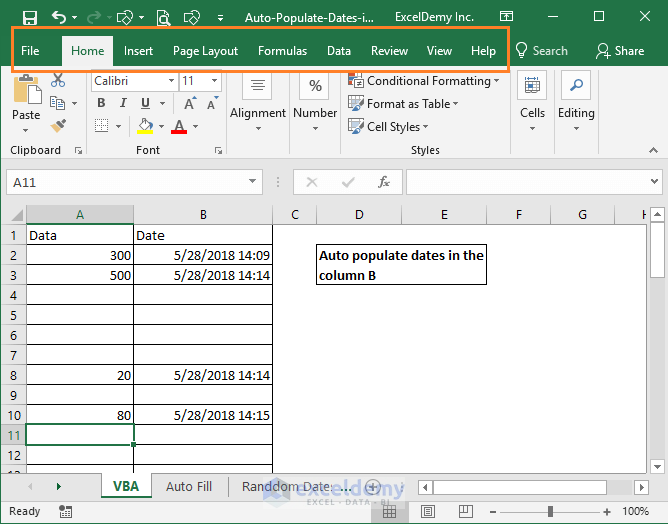2) Click on the File tab.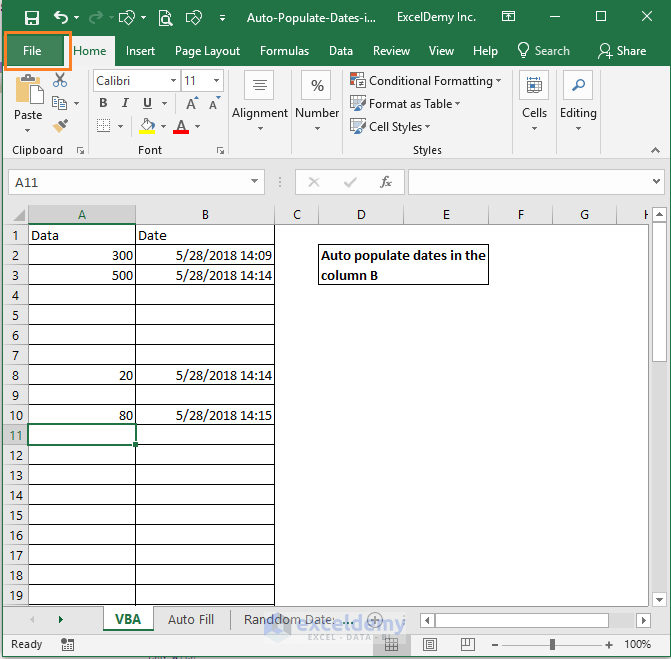3) Now choose Options from the left side menu.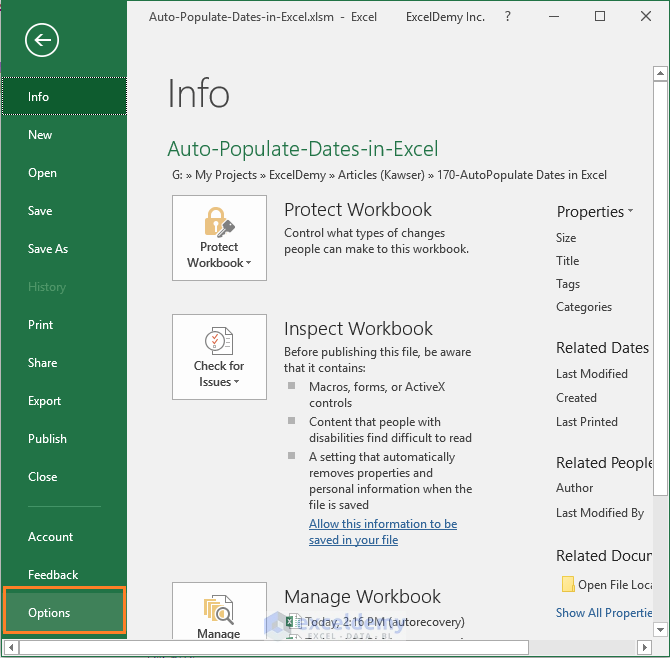4) Excel Options dialog box will appear. Choose Customize Ribbon and then on the right side of the dialog box, you see the Developer option is unchecked.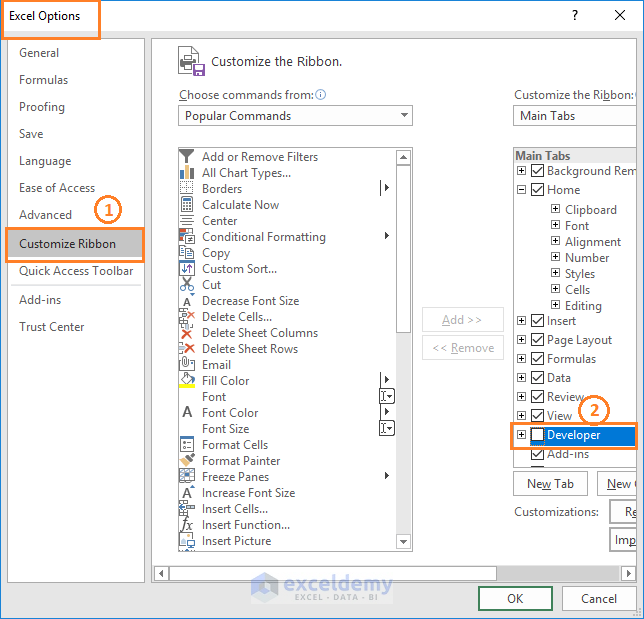5) Check the box and click OK on the dialog box.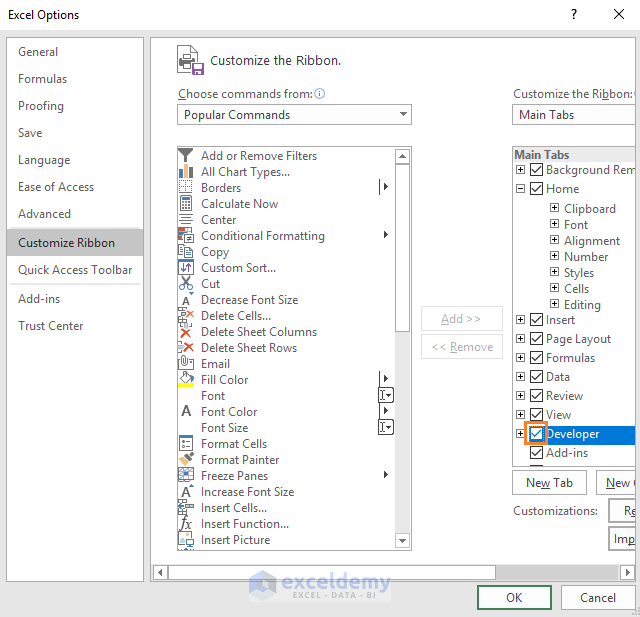6) You see, now the Developer tab is showing in the tab list.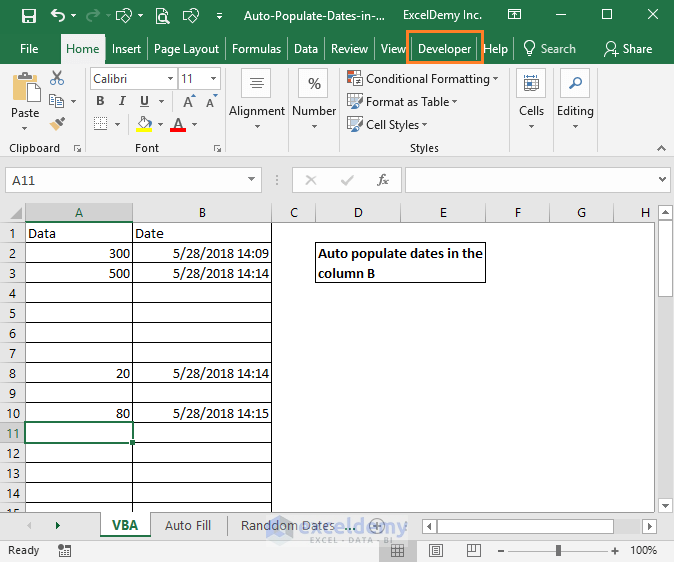So, these are two pieces of codes that you can use for your specific purposes. Do you know any better way to auto-populate dates in Excel when cells are updated? Let me know in the comment box.

Hello!
Welcome to my Excel blog! It took me some time to be a fan of Excel. But now I am a die-hard fan of MS Excel. I learn new ways of doing things with Excel and share here. Not only how to guide on Excel, but you will get also topics on Finance, Statistics, Data Analysis, and BI. Stay tuned!

1. ReplyGeorge Jun 20, 2018 at 10:03 PM

I altered the code to produce different results: Putting the Date in column E and the Time in column F. I am just wondering if there’s a better or shorter way to get this same results?

My revision:
Private Sub Worksheet_Change(ByVal Target As Excel.Range)
With Target
If .Count > 1 Then Exit Sub
If Not Intersect(Range(“D3:D22”), .Cells) Is Nothing Then
Application.EnableEvents = False
If IsEmpty(.Value) Then
.Offset(0, 1).ClearContents
Else
With .Offset(0, 1)
.NumberFormat = “dd mmm yyyy”
.Value = Now
End With
End If
Application.EnableEvents = True
End If
End With
‘Second Function ****
With Target
If .Count > 1 Then Exit Sub
If Not Intersect(Range(“D3:D22”), .Cells) Is Nothing Then
Application.EnableEvents = False
If IsEmpty(.Value) Then
.Offset(0, 2).ClearContents
Else
With .Offset(0, 2)
.NumberFormat = “hh:mm:ss”
.Value = Now
End With
End If
Application.EnableEvents = True
End If
End With
End Sub

• ReplyJun 21, 2018 at 12:28 PM

I will check out this code. Thanks for the input.

2. Replyanil Jan 28, 2019 at 2:56 AM

when we normally use as range object we also use set = “something here”. In the very first code above when we declare cell as range we did not use the set keyword. I did not understand why.Can you please explain it to me.

Private Sub Worksheet_Change(ByVal Target As Range)
‘ Auto Date
Dim Cell As Range

3. Replyammar May 1, 2019 at 11:13 PM

can I use 2 time stamps in the same excel sheet
input data in column “A” auto date in Column “C”
input data in column “K” auto date in Column “L”

code used:

Private Sub Worksheet_Change(ByVal Target As Range)
‘ Auto Date
Dim Cell As Range
For Each Cell In Target
If Cell.Column = Range(“D:D”).Column Then
If Cell.Value “” Then
Cells(Cell.Row, “B”).Value = Now
Else
Cells(Cell.Row, “B”).Value = “”
End If
End If
Next Cell
End Sub
End Sub

4. ReplyWayne Edmondson Jul 31, 2019 at 9:36 AM

Hi Kawser.. cool solution.. like your code. Another way to solve this is with a worksheet formula as follows:
=IF(A2=””,””,IF(B2″”,B2,NOW()))
The above assumes your data will go in column A, starting with A2 and the date/time stamp will go in column B, starting with B2. For this to work, you must enable Iterative Calculation in the Options=>Formulas=>Calculations options dialog. Just copy the formula down column B for as far as you want the stamp to work. While VBA can be more flexible, the above is a good way to get it done with a cell formula and an option change. I hope you like it. Thanks again for all of your tutorials.. super helpful and interesting.. makes me better at EXCEL, every time you send them. Thumbs up!

5. ReplyWayne Edmondson Jul 31, 2019 at 9:39 AM

Not sure what happened above to the formula. I think the editor can’t show the less than / greater than symbols. The formula should be:
=IF(A2=””,””,IF(B2 less than symbol and greater than symbol “”,B2,NOW()))
Hope that helps..

6. ReplyTori E Nov 3, 2019 at 4:43 AM

Is there a way to auto-populate a date based on a specific day rather than current day? For example auto-populate 60 days from August 28, 2019 rather than 60 days from current date?

7. ReplyVickie Watt Oct 31, 2020 at 10:32 AM

Thanks for always posting valuable info. I don’t know VBA, but in Excel NOW is volatile. If you insert it as described above, is the date static?